# Aldehydes, Ketones, Quinones and Derivatives

Quick move to entries starting with the letters C, D, H, K, Q or X.

acetals:*

Compounds having the structure R2C(OR')2 (R' not equal to H) and thus diethers of geminal diols. Originally, the term was confined to derivatives of aldehydes (one R = H), but now it applies equally to derivatives of ketones (neither R = H). NOC Rule C-331, GNOC Recom. R-5.6.4.1. Mixed acetals have different R' groups. See also acetonides, ketals. Cf. acylals, hemiacetals.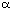-Hydroxy ketones, RCH(OH)C(=O)R. NOC Rule C-333. So named from the fact that they are formally derived from reductive coupling of carboxylic acyl groups. See also ketones.

Compounds RC(=O)H, in which a carbonyl group is bonded to one hydrogen atom and to one R group. NOC Rule C-301.1.

1. Compounds containing the carbonyl group, C=O. The term is commonly used in the restricted sense of aldehydes and ketones, although it actually includes carboxylic acids and derivatives. See under oxo compounds.

2. Metal carbonyls, in which carbon monoxide is a formal ligand.

Cyclic-diketones, which exist predominantly in an enolic form.

1,3-Diphenylbut-2-en-1-one [PhC(=O)CH=C(CH3)Ph] and its ring-substituted derivatives.

Compounds having the general formula R2C(OH)OR' (R' not equal to H). NOC Rule C-331.4. GNOC Recom. R-5.6.4.2. See also lactols, hemiketals.

Hemiacetals having the structure R2C(OH)OR (R not equal to H), derived from ketones by formal addition of an alcohol to the carbonyl group. This term, once abandoned (NOC Rule C-331.4, footnote) has been reinstated as a subclass of hemiacetals. GNOC Recom. R-5.6.4.2.

Acetals derived from ketones by replacement of the oxo group by two hydrocarbyloxy groups: R2C(OR)2 (R not equal to H). This term, once abandoned (NOC Rule C-333.1), has been reinstated (GNOC Recom. R-5.6.4.1) as a subclass of acetals.

Compounds in which a carbonyl group is connected by a double bond to an alkylidene group: R2C=C=O. NOC Rule C-321.

keto: see under oxo compounds.

Compounds in which a carbonyl group is bonded to two carbon atoms: R2C=O (neither R may be H). NOC Rule C-311.1. Note: Compounds of structure such as R3SiC(=O)R are not ketones but acyl derivatives of substituted silanes.

Molecular complexes of one equivalent amount of a quinone with one equivalent amount of the corresponding hydroquinone.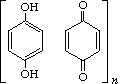Methylidenecyclohexadienones and dimethylidenecyclohexadienes, formally derived from quinones by replacement of one or both of the quinone oxygens by methylidene groups. (The diradical, triplet state of quinodimethanes can also be called o- or p-xylylenes).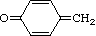p-quinomethane,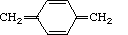p-quinodimethane

quinomethides/quinone methides: Undesirable names for quinomethanes.

Compounds having a fully conjugated cyclic dione structure, such as that of benzoquinones, derived from aromatic compounds by conversion of an even number of -CH= groups into -C(=O)- groups with any necessary rearrangement of double bonds (polycyclic and heterocyclic analogues are included). NOC Rule C-317.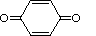p-benzoquinone

xylylenes: See under quinomethanes.